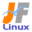Linux Kernel 2.4 Documentation: /usr/src/linux/Documentation/binfmt_misc.txt

binfmt_misc.txt

\$B?'!9\$J%P%\$%J%j7A<0\$N%+!<%M%k\$K\$h\$k%5%]!<%H(B [\$B%W%l%\$%s%F%-%9%HHG(B]
• \$B86Cx:n
• \$BK]Lu
• \$B%P!<%8%g%s(B: 2.4.0
• \$BK]LuF|;~(B: 1999/05/08
```

\$B\$\$\$m\$s\$J(B (\$B\$*9%\$_\$N(B) \$B%P%\$%J%j%U%)!<%^%C%H\$N%+!<%M%k%5%]!<%H(B v1.1

=====================================================================

\$B\$3\$N%+!<%M%k\$G\$O%7%'%k\$+\$i\$=\$NL>A0\$r%?%\$%W\$9\$k\$@\$1\$G\$[\$H\$s\$I\$N%W%m%0%i(B
\$B%`\$r(B(\$B@)8B\$O2<\$r;2>H(B) \$B5/F0\$9\$k\$3\$H\$,\$G\$-\$^\$9!#\$3\$NCf\$K\$ONc\$(\$P(B Java(TM)\$B!"(B
Python\$B!"(BEmacs \$B%W%m%0%i%`\$,4^\$^\$l\$^\$9!#(B

\$B\$3\$l\$,\$G\$-\$k\$h\$&\$K\$9\$k\$K\$O!"%P%\$%J%j\$H0l=o\$K%\$%s%?!<%W%j%?\$,5/F0\$5\$l\$k(B
\$B\$h\$&\$K(Bbinfmt_misc \$B\$K65\$(\$F\$d\$i\$J\$1\$l\$P\$\$\$1\$^\$;\$s!#(BBinfmt_misc \$B\$O%U%!%\$(B
\$B%k\$N@hF,\$N2?%P%\$%H\$+\$r\$"\$J\$?\$N;XDj\$7\$?%^%8%C%/%P%\$%H%7!<%1%s%9\$H(B(\$BFCDj(B
\$B\$N%S%C%H\$O%^%9%/\$7\$F(B)\$BHf3S\$7!"%P%\$%J%j%?%\$%W\$rH=Dj\$7\$^\$9!#(BBinfmt_misc
\$B\$O%U%!%\$%kL>\$N3HD%;R!"\$D\$^\$j(B \$B!V(B.com\$B!W(B \$B\$d(B \$B!V(B.exe\$B!W\$bG'<1\$9\$k\$3\$H\$,\$G\$-(B
\$B\$^\$9!#(B

\$B?7\$7\$\$%P%\$%J%j%?%\$%W\$rEPO?\$9\$k\$K\$O!"A0\$G:n\$i\$l\$^\$9!#(B

- type \$B\$OG'<1\$N%?%\$%W\$G\$9!#%^%8%C%/\$N>l9g\$O(B M\$B!"3HD%;R\$N>l9g\$O(B E \$B\$r;X(B
\$BDj\$7\$F2<\$5\$\$!#(B

\$B\$k\$H(B (\$B\$D\$^\$j(B \$B!V(B:name:type::magic...\$B!W(B \$B\$H=q\$/\$H(B)\$B%G%U%)%k%H\$N(B 0 \$B\$K\$J(B
\$B\$j\$^\$9!#(B

- magic \$B\$O(B binfmt_misc \$B\$,%^%C%A%s%0\$K;H\$&%P%\$%H%7!<%1%s%9\$G\$9!#%^%8%C(B
\$B%/J8;zNs\$NCf\$K\$O(B \x0a \$B\$d(B \xA4 \$B\$N\$h\$&\$J(B16\$B?J?t\$NJ8;z\$r4^\$a\$k\$3\$H\$,\$G(B
\$B\$-\$^\$9!#(B
\$B%7%'%k\$N4D6-\$G\$O!"%7%'%k\$,(B \ \$B\$r?)\$C\$F\$7\$^\$o\$J\$\$\$h\$&\$K(B \\x0a \$B\$H=q\$+(B
\$B\$J\$1\$l\$P\$\$\$1\$^\$;\$s!#(B
\$B\$b\$7%U%!%\$%kL>3HD%;R\$K\$h\$k%^%C%A%s%0\$rA*\$s\$@\$N\$J\$i!"\$3\$3\$OG'<1\$5\$l(B
\$B\$k\$Y\$-3HD%;R\$K\$J\$j\$^\$9(B (\$B%T%j%*%I!V(B.\$B!W\$OITMW!#(B\x0a \$B\$N\$h\$&\$JI=5-\$O\$G(B
\$B\$-\$^\$;\$s(B)\$B!#(B
\$B3HD%;R\$K\$h\$k%^%C%A%s%0\$OBgJ8;z>.J8;z\$r6hJL\$7\$^\$9!*(B

\$BF1MM\$NJ8;zNs\$r;XDj\$9\$k\$3\$H\$G!"FCDj\$N%S%C%H\$r%^%C%A%s%0\$+\$i30\$9\$3\$H(B
\$B\$,\$G\$-\$^\$9!#(B
\$B%^%9%/\$O%U%!%\$%k\$N%P%\$%HNs\$H(B AND \$B1i;;\$5\$l\$^\$9!#(B

- interpreter \$B\$O%P%\$%J%j\$r:G=i\$N0z?t\$H\$7\$F5/F0\$5\$l\$k\$Y\$-%W%m%0%i%`\$G\$9(B
(\$B%U%k%Q%9\$G;XDj(B)\$B!#(B

\$B\$\$\$/\$D\$+\$N@)8B\$,\$"\$j\$^\$9!#(B

- \$B@_DjJ8;zNs\$N%H!<%?%k\$O(B 255 \$BJ8;z\$rD6\$(\$F\$O\$\$\$1\$^\$;\$s(B

- \$B%^%8%C%/\$O%U%!%\$%k\$N:G=i\$N(B 128 \$B%P%\$%H0JFb\$K0LCV\$7\$J\$1\$l\$P\$\$\$1\$^\$;\$s!#(B
\$B\$9\$J\$o\$A!"(Boffset+size(magic) \$B\$,(B 128 \$B\$h\$j>.\$5\$/\$J\$1\$l\$P\$\$\$1\$^\$;\$s!#(B

- \$B%\$%s%?%W%j%?J8;zNs\$O(B 127 \$BJ8;z\$rD6\$(\$F\$O\$\$\$1\$^\$;\$s(B

\$B%V!<%H;~\$K(B /etc/rc \$B%9%/%j%W%H\$NCf\$G%P%\$%J%j%U%)!<%^%C%H\$rDI2C\$7\$?\$\$\$3(B
\$B\$H\$,\$"\$k\$+\$bCN\$l\$^\$;\$s!#\$3\$l\$r@5\$7\$/9T\$&J}K!\$r8+\$D\$1\$k\$K\$O\$"\$J\$?\$N(B
init \$B%W%m%0%i%`\$N%^%K%e%"%k\$rFI\$s\$G2<\$5\$\$!#(B

\$B%(%s%H%j\$rDI2C\$9\$k=gHV\$KCm0U\$7\$F2<\$5\$\$!*(B \$B8e\$+\$iDI2C\$7\$?%(%s%H%j\$,@h\$K(B
\$B%^%C%A%s%0\$5\$l\$^\$9!#(B

\$BNc\$r<(\$7\$^\$9(B(/proc/sys/fs/binfmt_misc \$B%G%#%l%/%H%j\$K\$\$\$k\$b\$N\$H2>Dj\$7\$F(B
\$B\$\$\$^\$9(B)\$B!#(B

- em86 \$B\$r%5%]!<%H\$9\$k(B(binfmt_em86 \$B\$N\$h\$&\$J\$b\$N!#(BAlpha AXP \$B@lMQ(B)
echo
':i386:M::\x7fELF\x01\x00\x00\x00\x00\x00\x00\x00\x00\x00\x00\x00\x02\x00\x03:\xff\xff\xff\xff\xff\xfe\xfe\xff\xff\xff\xff\xff\xff\xff\xff\xff\xfb\xff\xff:/bin/em86:'
> register
echo
':i486:M::\x7fELF\x01\x00\x00\x00\x00\x00\x00\x00\x00\x00\x00\x00\x02\x00\x06:\xff\xff\xff\xff\xff\xfe\xfe\xff\xff\xff\xff\xff\xff\xff\xff\xff\xfb\xff\xff:/bin/em86:'
> register

- \$B%Q%C%/\$5\$l\$?(B DOS \$B%"%W%j%1!<%7%g%s\$r%5%]!<%H\$9\$k(B (\$B%W%l%3%s%U%#%.%e%"(B
\$B%I(B dosemu hd \$B%\$%a!<%8(B)
echo ':DEXE:M::\x0eDEX::/usr/bin/dosexec:' > register

- wine \$B\$r;H\$C\$F(B Windows \$B\$NuBV\$,\$o\$+\$j\$^\$9!#(B

\$B\$"\$k%(%s%H%j\$^\$?\$O\$9\$Y\$F\$N%(%s%H%j\$r:o=|\$9\$k\$?\$a\$K\$O!"(B/proc/.../the_name
\$B\$^\$?\$O(B /proc/sys/fs/binfmt_misc/status \$B\$K(B -1  \$B\$r%(%3!<\$7\$^\$9!#(B

\$B%R%s%H(B
======

\$B%\$%s%?%W%j%?\$KFCJL\$J0z?t\$rEO\$7\$?\$\$;~\$O!"\$=\$N\$?\$a\$N%i%C%Q!<%9%/%j%W%H(B
(wrapper script) \$B\$r=q\$/\$3\$H\$,\$G\$-\$^\$9!#%5%s%W%k\$O(B Documentation/java.txt
\$B\$r8+\$F2<\$5\$\$!#(B

\$B\$"\$J\$?\$N%\$%s%?%W%j%?\$O%U%!%\$%kL>\$N(B PATH \$B\$r;2>H\$7\$F\$O!V\$\$\$1\$^\$;\$s!W!#(B
\$B%+!<%M%k\$O%U%k%U%!%\$%kL>\$rEO\$7\$F\$\$\$^\$9!#(BPATH \$B\$r;H\$&\$HM=4|\$;\$LF0:n\$r(B
\$B\$7\$?\$j%;%-%e%j%F%#E*\$K4m81\$J\$3\$H\$,\$"\$j\$^\$9!#(B

binfmt_misc \$B\$K4X\$9\$k%Z!<%8\$O(B
http://www.anatom.uni-tuebingen.de/~richi/linux/binfmt_misc.html
\$B\$G\$9!#(B

Richard G\$B!&(Bther, richard.guenther@student.uni-tuebingen.de

\$BF|K\8lLu!'NS(B(hayashi884@geocities.co.jp) 1999.5.8 (Ver.0.1)
-----------------------------------------------------------
(\$B0J2<86J8(B)

Kernel Support for miscellaneous (your favourite) Binary Formats v1.1
=====================================================================

This Kernel feature allows you to invoke almost (for restrictions see below)
every program by simply typing its name in the shell.
This includes for example compiled Java(TM), Python or Emacs programs.

To achieve this you must tell binfmt_misc which interpreter has to be invoked
with which binary. Binfmt_misc recognises the binary-type by matching some bytes
at the beginning of the file with a magic byte sequence (masking out specified
bits) you have supplied. Binfmt_misc can also recognise a filename extension
aka '.com' or '.exe'.

To actually register a new binary type, you have to set up a string looking like
:name:type:offset:magic:mask:interpreter: (where you can choose the ':' upon
your needs) and echo it to /proc/sys/fs/binfmt_misc/register.
Here is what the fields mean:
- 'name' is an identifier string. A new /proc file will be created with this
name below /proc/sys/fs/binfmt_misc
- 'type' is the type of recognition. Give 'M' for magic and 'E' for extension.
- 'offset' is the offset of the magic/mask in the file, counted in bytes. This
defaults to 0 if you omit it (i.e. you write ':name:type::magic...')
- 'magic' is the byte sequence binfmt_misc is matching for. The magic string
may contain hex-encoded characters like \x0a or \xA4. In a shell environment
you will have to write \\x0a to prevent the shell from eating your \.
If you chose filename extension matching, this is the extension to be
recognised (without the '.', the \x0a specials are not allowed). Extension
matching is case sensitive!
bits from matching by supplying a string like magic and as long as magic.
The mask is anded with the byte sequence of the file.
- 'interpreter' is the program that should be invoked with the binary as first
argument (specify the full path)

There are some restrictions:
- the whole register string may not exceed 255 characters
- the magic must reside in the first 128 bytes of the file, i.e.
offset+size(magic) has to be less than 128
- the interpreter string may not exceed 127 characters

You may want to add the binary formats in one of your /etc/rc scripts during
boot-up. Read the manual of your init program to figure out how to do this
right.

A few examples (assumed you are in /proc/sys/fs/binfmt_misc):

- enable support for em86 (like binfmt_em86, for Alpha AXP only):
echo ':i386:M::\x7fELF\x01\x00\x00\x00\x00\x00\x00\x00\x00\x00\x00\x00\x02\x00\x03:\xff\xff\xff\xff\xff\xfe\xfe\xff\xff\xff\xff\xff\xff\xff\xff\xff\xfb\xff\xff:/bin/em86:' > register
echo ':i486:M::\x7fELF\x01\x00\x00\x00\x00\x00\x00\x00\x00\x00\x00\x00\x02\x00\x06:\xff\xff\xff\xff\xff\xfe\xfe\xff\xff\xff\xff\xff\xff\xff\xff\xff\xfb\xff\xff:/bin/em86:' > register

- enable support for packed DOS applications (pre-configured dosemu hdimages):
echo ':DEXE:M::\x0eDEX::/usr/bin/dosexec:' > register

- enable support for Windows executables using wine:
echo ':DOSWin:M::MZ::/usr/local/bin/wine:' > register

For java support see Documentation/java.txt

You can enable/disable binfmt_misc or one binary type by echoing 0 (to disable)
or 1 (to enable) to /proc/sys/fs/binfmt_misc/status or /proc/.../the_name.
Catting the file tells you the current status of binfmt_misc/the entry.

You can remove one entry or all entries by echoing -1 to /proc/.../the_name
or /proc/sys/fs/binfmt_misc/status.

HINTS:
======

If you want to pass special arguments to your interpreter, you can
write a wrapper script for it. See Documentation/java.txt for an
example.

Your interpreter should NOT look in the PATH for the filename; the
kernel passes it the full filename to use.  Using the PATH can cause
unexpected behaviour and be a security hazard.

There is a web page about binfmt_misc at
http://www.anatom.uni-tuebingen.de/~richi/linux/binfmt_misc.html

Richard Gther, richard.guenther@student.uni-tuebingen.de
```